CBSE Class 12 Sample Paper for 2020 Boards

Class 12
Solutions of Sample Papers and Past Year Papers - for Class 12 Boards

##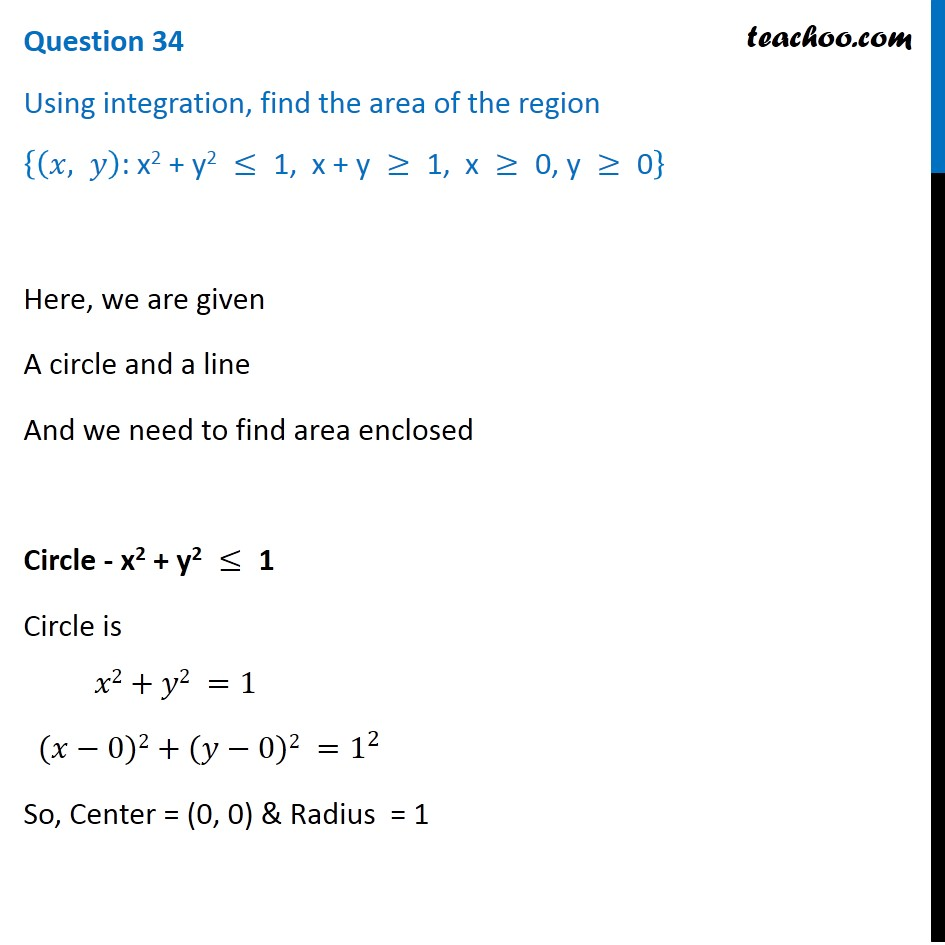googletag.cmd.push(function() { googletag.display('div-gpt-ad-1669298377854-0'); });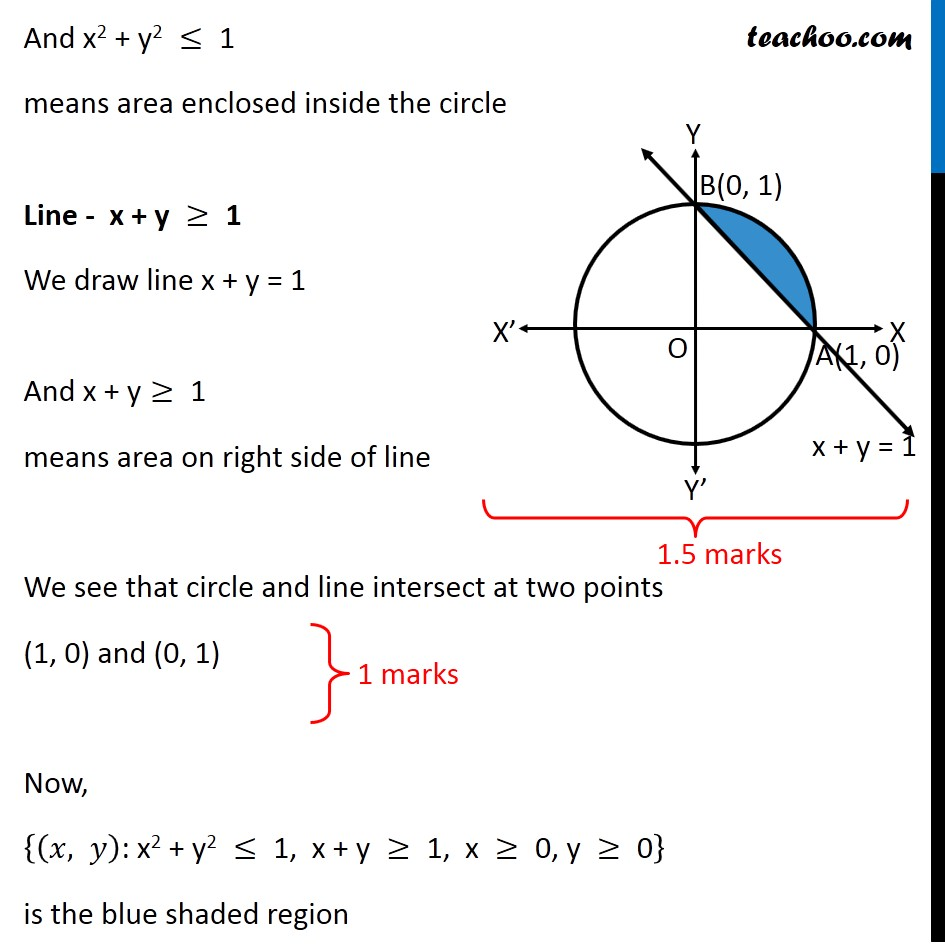(adsbygoogle = window.adsbygoogle || []).push({});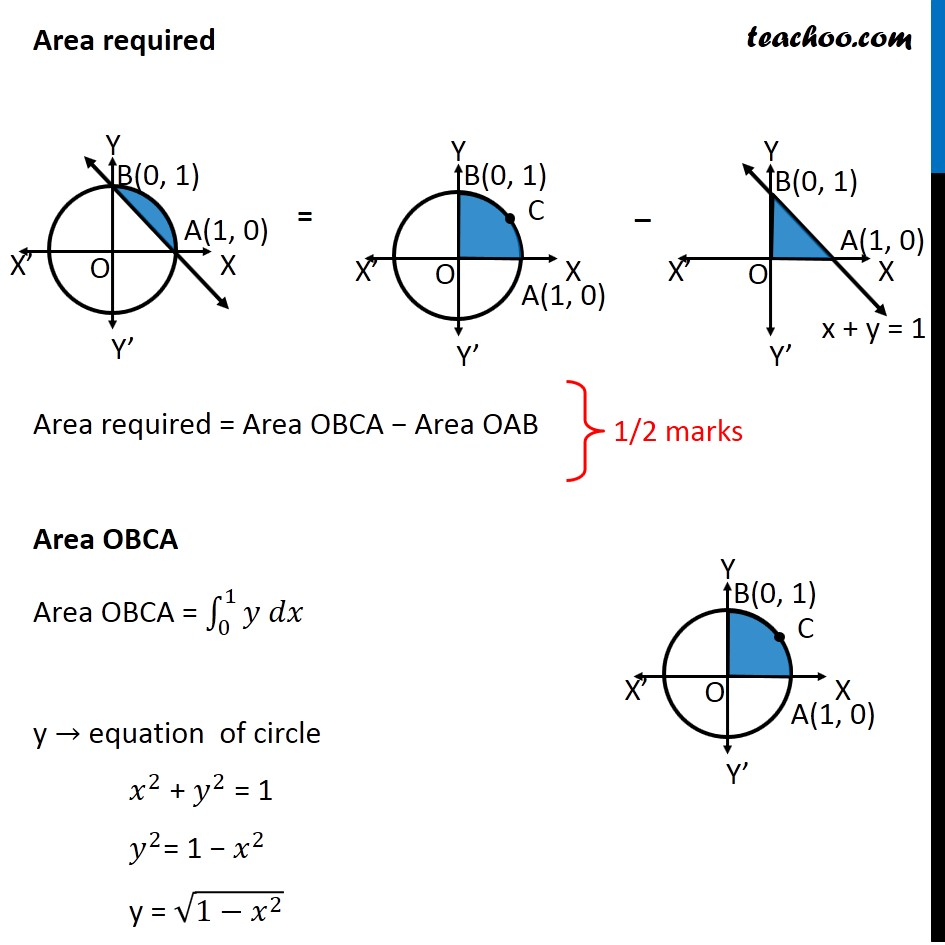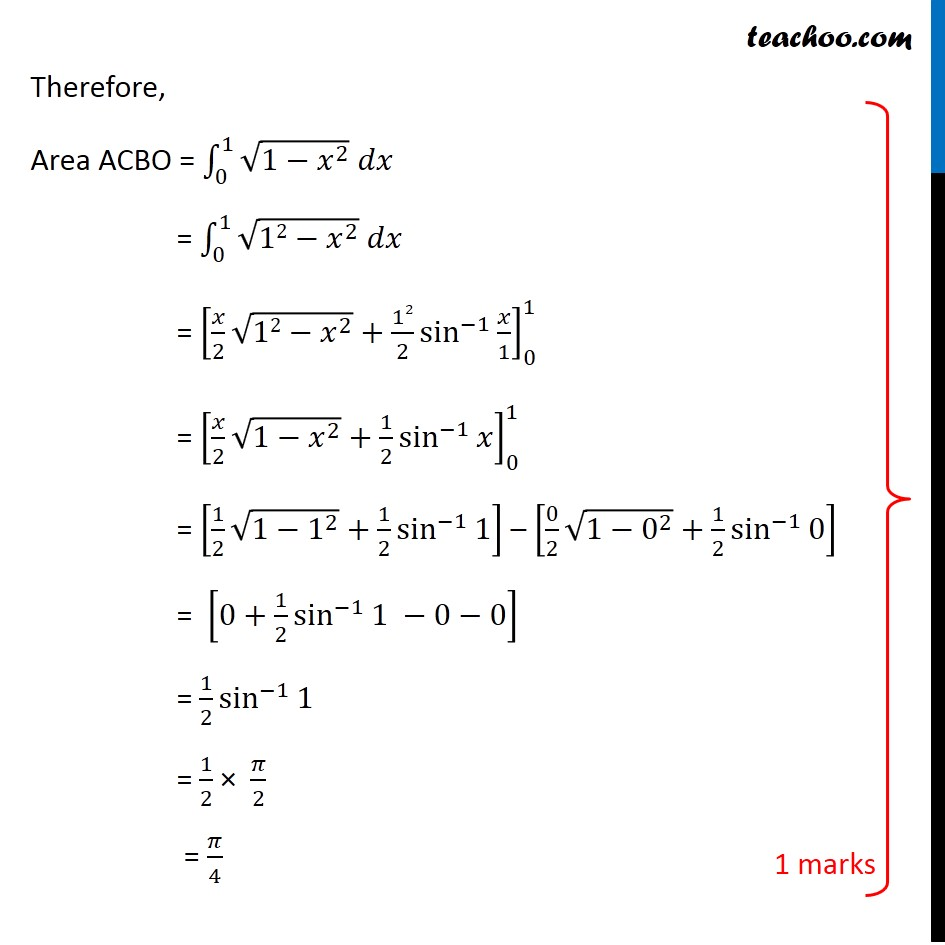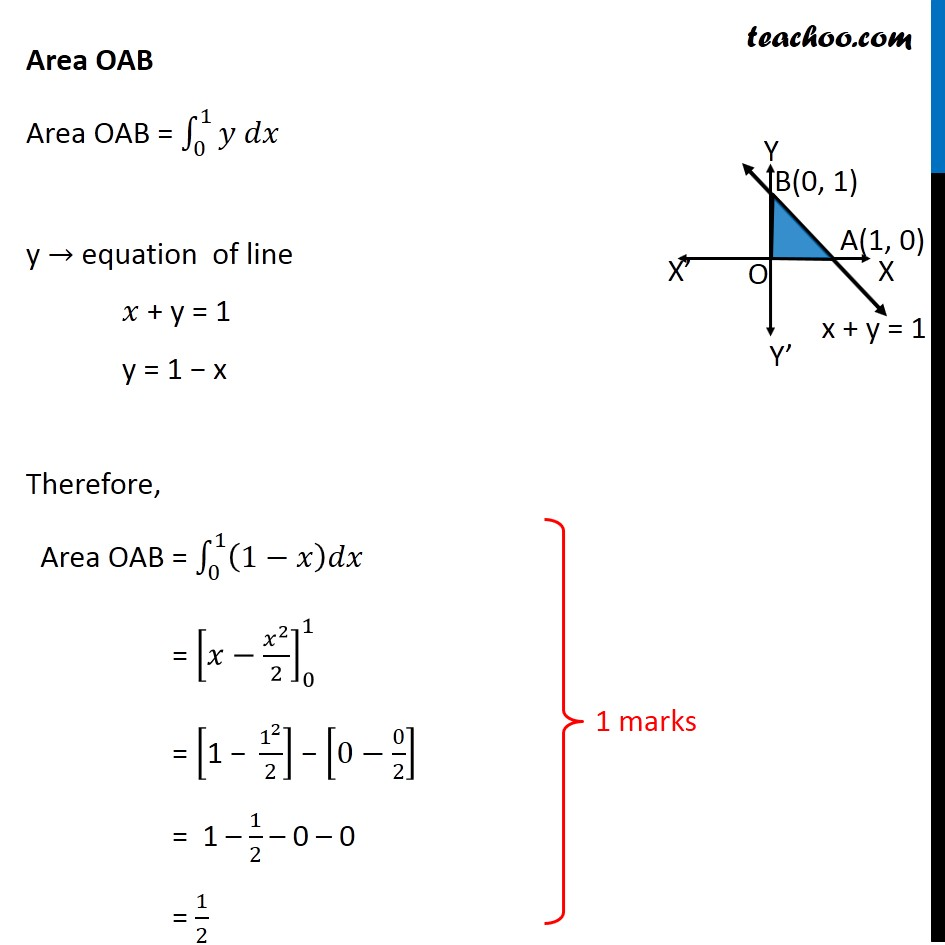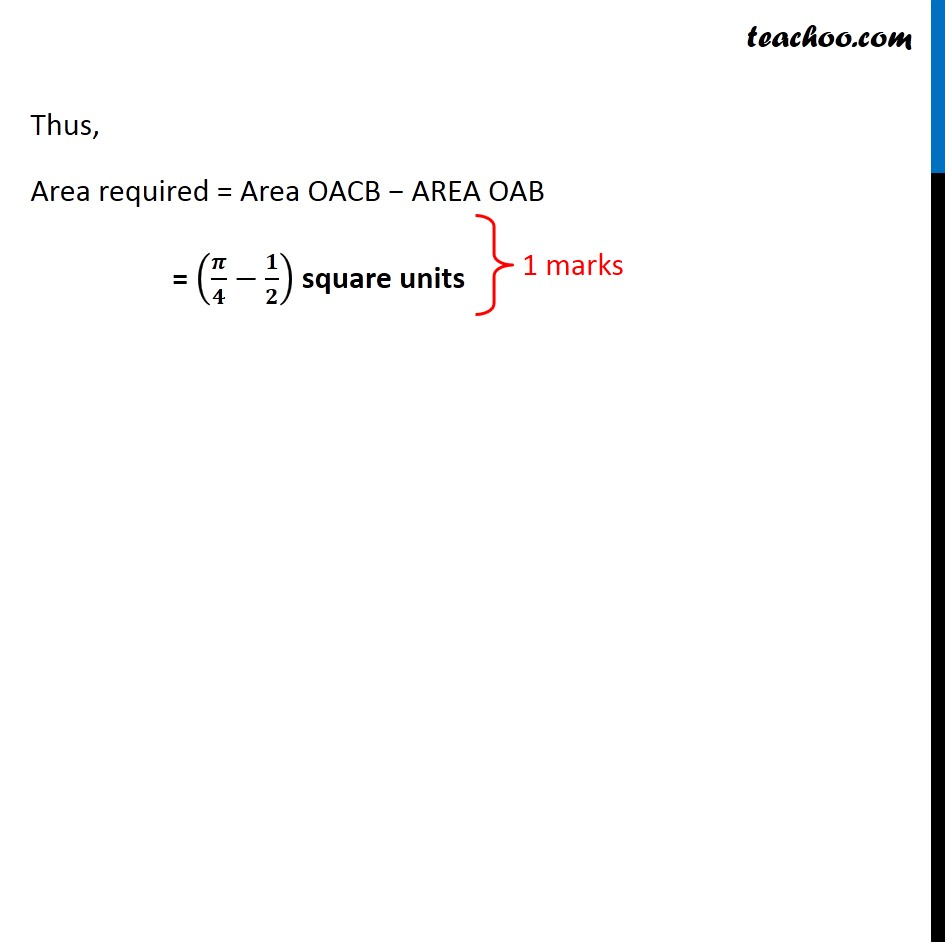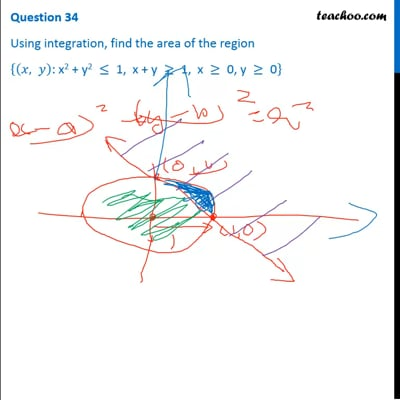This video is only available for Teachoo black users

Learn in your speed, with individual attention - Teachoo Maths 1-on-1 Class

### Transcript

Question 34 Using integration, find the area of the region {(𝑥, 𝑦)": x2 + y2 " ≤" 1, x + y " ≥" 1, x " ≥" 0, y " ≥" 0" } Here, we are given A circle and a line And we need to find area enclosed Circle - "x2 + y2 "≤" 1" Circle is 𝑥2+𝑦2 =1 (𝑥−0)2+(𝑦−0)2 =1^2 So, Center = (0, 0) & Radius = 1 And "x2 + y2 "≤" 1" means area enclosed inside the circle Line -" x + y "≥" 1" We draw line x + y = 1 And "x + y"≥" 1" means area on right side of line We see that circle and line intersect at two points (1, 0) and (0, 1) Now, {(𝑥, 𝑦)": x2 + y2 " ≤" 1, x + y " ≥" 1, x " ≥" 0, y " ≥" 0" } is the blue shaded region Area required Area required = Area OBCA − Area OAB Area OBCA Area OBCA = ∫1_0^1▒〖𝑦 𝑑𝑥〗 y → equation of circle 𝑥^2 + 𝑦^2 = 1 𝑦^2= 1 − 𝑥^2 y = √(1−𝑥^2 ) Therefore, Area ACBO = ∫1_0^1▒〖√(1−𝑥^2 ) " " 𝑑𝑥〗 = ∫1_0^1▒〖√(12−𝑥^2 ) 𝑑𝑥〗 = [𝑥/2 √(12−𝑥^2 )+12/2 sin^(−1)⁡〖𝑥/1〗 ]_0^1 = [𝑥/2 √(1−𝑥^2 )+1/2 sin^(−1)⁡𝑥 ]_0^1 = [1/2 √(1−1^2 )+1/2 sin^(−1)⁡1 ] – [0/2 √(1−0^2 )+1/2 sin^(−1)⁡0 ] = [0+1/2 sin^(−1)⁡1 −0−0] = 1/2 sin^(−1)⁡1 = 1/2 × 𝜋/2 = 𝜋/4 Area OAB Area OAB = ∫1_0^1▒〖𝑦 𝑑𝑥〗 y → equation of line 𝑥 + y = 1 y = 1 − x Therefore, Area OAB = ∫1_0^1▒(1−𝑥)𝑑𝑥 = [𝑥−𝑥^2/2]_0^1 = ["1 − " 1^2/2] − [0−0/2] = 1 – 1/2 – 0 – 0 = 1/2 Thus, Area required = Area OACB − AREA OAB = (𝝅/𝟒−𝟏/𝟐) square units